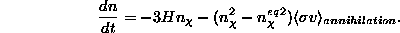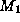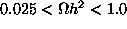The Net Advance of Physics: The Nature of Dark Matter, by Kim Griest -- Section 6C.

Next: Accelerator Constraints Up: Search for Wimps Previous: Motivation for Supersymmetry

## Relic Abundance in More Detail

The number density of any particle which was once in thermal

equilibrium in the Early Universe can be found by solving the

relevant set of Boltzmann equations. In most cases only one is

needed:where H is the Hubble constant, n is the number density, t is time,

andis the thermally averaged cross section times the relative

velocity of the interacting particles. We are usingto denote the

LSP. The first term on the right-hand side is the reduction in LSP

number density due to the Hubble expansion, the second term is

reduction due to self-annihilation, and the third term is the

increase due to particle production. The third term can be

simplified using the fact that ``ordinary" particles such as quarks

and electrons stay in thermal equilibrium throughout the period

during which the Wimp number density ``freezes out" (see Section

4). When thermal equilibrium obtains, creation equals

annihilation, so the second and third terms are equal. Therefore

one can eliminate the ``ordinary particle" cross sections and

number densities and find the usual equationStarting at an early time when all particles were in equilibrium, one

integrates this equation either numerically or using the standard

``freeze-out" approximation , and obtains the number density

at t=0 (today). The relic abundance is simply,

whereis the mass of the LSP.

The difficult step in obtaining the current day density is usually the

calculation of the annihilation cross section of two LSPs into all

standard model particles. In order to perform this calculation, one

must first determine which particle is the LSP, and then evaluate all

the relevant Feynman diagrams. Going through the list of

supersymmetric particles, one finds, basically by process of

elimination, that only the sneutrino and neutralino are likely

candidates. In the vast majority of models, the neutralino is favored

over the sneutrino, so most work has concentrated on the

neutralino as dark matter candidate.

For the neutralino, several dozen Feynman diagrams contribute to

self-annihilation, including possible annihilation into quarks,

leptons, W, Z, and Higgs bosons, and involving most of the

super-partners as exchange particles. So in order to perform the

calculation one needs to first obtain the mass and couplings of all

the supersymmetric particles. Since supersymmetry is broken, the

mass terms are unknown, giving rise to many free parameters in the

most general supersymmetric model. Usually, in order to simplify

things, one considers the ``minimal" supersymmetric model,

the model with the fewest new particles, but still there are many

undetermined parameters. So to further simplify, several other

In minimal supergravity, some GUT scale assumptions can reduce

the number of parameters to just a few. In what follows, we use

some, but not all, of the supergravity assumptions, and have as a

result 5 free parameters . The parameters are the gaugino mass

parametersand, the Higgsino mass parameter, the

pseudoscalar Higgs mass, and the ratio of Higgs vacuum

expectation values. For any set of parameters, one can

calculate all the masses, mixings, and couplings, and then the

annihilation cross section. Thus after a long calculation, one finally

obtainsin terms of the five parameters. If one obtains a relic

abundance in the range, then that set of

parameters defines a potential dark matter candidate. However,

before deciding that this is a dark matter candidate one must ensure

that one of the many accelerator experiments that have searched

for supersymmetric particles has not already ruled out that model.Next: Accelerator Constraints Up: Search for Wimps Previous: Motivation for Supersymmetry